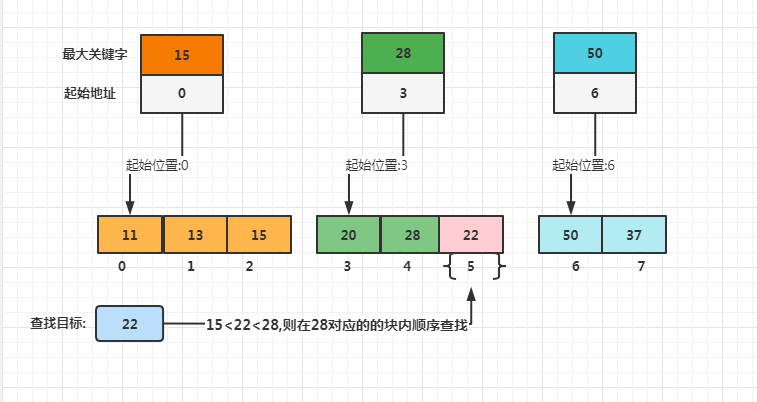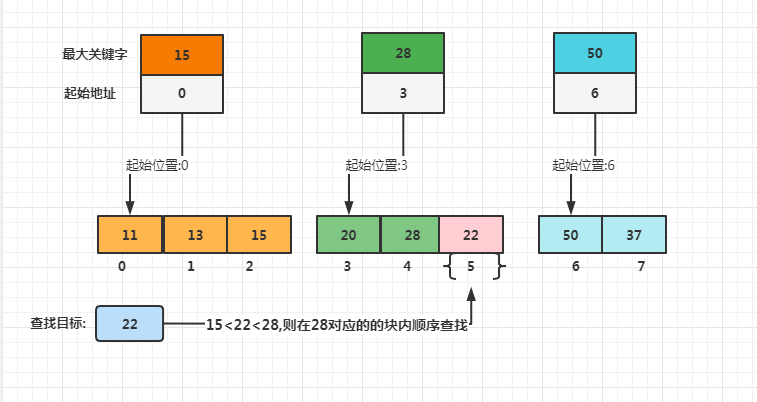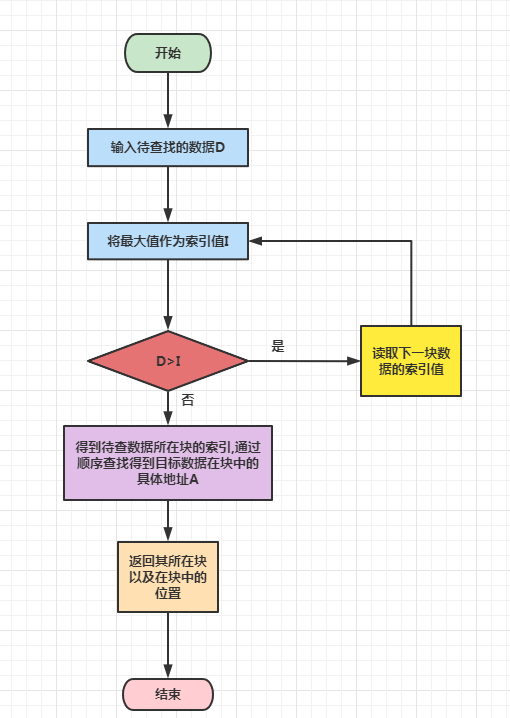# 【算法实践】分块查找知多少？手把手带你实现分块查找

• 2022 年 9 月 18 日
广东
• 本文字数：2070 字

阅读完需：约 7 分钟# 图解分块查找

## 分块查找设计

### 查找图示### 查找过程

1. 第一步是在索引表中确定待查记录所在的块，可以顺序查找或折半查找索引表

2. 第二步是在块内顺序查找# 代码实现

1. 输入待查的目标数据

2. 把最大值作为索引值

3. 判断待查数据是否大于索引值,如果大于索引值,那么比较下一块数据的索引值,否则得到当前当前待查找数据所在块的索引

4. 通过顺序查找得到待查找数据在块中的位置

5. 返回目标数据的位置

Length = 8 #输入元素的个数targets = 0pos = -1tabNum = 3 #分块tabPos = -1print("请输入列表，以空格键隔开数字:",end=' ')list=[int(s) for s in input().split()]goal = int(input('请输入待查元素：'))   #用户输入查找值print('需要在列表中在找到：', goal)

list_index = []  #使用二维列表表示多个子序列for i in range(tabNum):  # 在列表中添加m个列表    list_index.append([])for i in range(1, tabNum):      list_index[i].append(list[i - 1])  for i in range(1, tabNum - 1):  # 将添加元素的子列表中的元素降序排列    for j in range(1, tabNum - i):        if list_index[j] < list_index[j + 1]:            list_index[j], list_index[j + 1] = list_index[j + 1], list_index[j]for i in range(tabNum - 1, Length):      for j in range(1, tabNum):        if list[i] > list_index[j]:            list_index[j - 1].append(list[i])            break    else:        list_index[tabNum - 1].append(list[i])if len(list_index) > 1:      for i in range(len(list_index) - 1, 0, -1):        if list_index[i] > list_index[i - 1]:            list_index[i], list_index[i - 1] = list_index[i - 1], list_index[i]print("子列表结构：",list_index)  # 输出子列表

for i in range(tabNum - 1, -1, -1):  #确定给定元素位置    if len(list_index[i]) != 0 and goal < list_index[i]:        for j in range(len(list_index[i])):            if list_index[i][j] == goal:                tabPos = i + 1                pos = j + 1                targets = 1if targets:    print("在第", tabPos,"个子列表中第",pos,"的位置")else:    print("没找到")# 总结## 评论